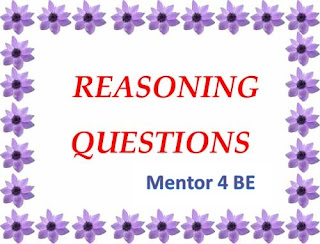## Reasoning Quiz for Bank Exams - IBPS/BOBMentor For Bank Exams
Welcome to Mentor for Bank Exams. Here I am presenting Reasoning Quiz on Three Digit Numbers based on the new pattern of bank exams like SBI PO, IBPS PO and other exams. Candidates who are preparing for upcoming exams can make use of these. Please write your suggestions and quires in the comment box below.
Directions (1 – 5): There are five three digit numbers are given below. Answer the questions based on them.
910        989        221        632        236
1. If the first and the third digit of all the numbers are interchanged, then the sum of the largest and the smallest number is
a) 929
b) 1111
c) 343
d) 1008
e) 1210
2. Product of last two digits of the second lowest number is
a) 18
b) 8
c) 12
d) 4
e) 6
3. For all the numbers, if the second digit is increased by 1 and the first digit is decreased by 2 and then all the three digits are reversed. The difference between the largest and the smallest number formed is
a) 768
b) 970
c) 867
d) 966
e) 753
4. What is the sum of the second and the third digits of the third lowest number?
a) 9
b) 6
c) 10
d) 4
e) 5
5. If the first and the second digits of all the numbers is interchanged, then the product of the second and the third digits of the second highest number is
a) 18
b) 0
c) 12
d) 72
e) 2
Directions (6 – 10): Following questions are based on the five three digit numbers given below:
823        634        768        523        972
6. If the first and the second digits of all the numbers are interchanged, then the highest value of the product of the second and the third digit is
a) 56
b) 24
c) 15
d) 18
e) 32
7. If all the digits are arranged in increasing order for all the numbers, then the sum of the second and the third digit for the third highest number is
a) 18
b) 16
c) 14
d) 12
e) 10
8. For all the numbers, if the third digit is decreased by 2, then the highest value of the sum of the first and the third digit is
a) 13
b) 9
c) 15
d) 21
e) 18
9. If all the numbers are arranged in descending order then the sum of the second and the third digit of the third lowest number is
a) 11
b) 9
c) 17
d) 14
e) 19
10. If the sum of the first and the second digit of all the numbers is arranged in increasing order, then what will be the second lowest such sum?
a) 7
b) 8
c) 9
d) 10
e) 11
1)d   2)a   3)b   4)e   5)c   6)a   7)b   8)a   9)d   10)c
Solutions:
1. D)
After interchanging the digits, the smallest number is 19 and the largest number is 989.
Hence the sum is 19 + 989 = 1008
2. A)
Second lowest number is 236
Hence the product of last two digits of 236 = 3 x 8 = 18
3. B)
If the second digit is increased by 1 and first digit decreased by 2, then the numbers are
720        799        031        442        046
And after reversing the digits the numbers are 027              997        130        224        640
Largest number is 997 and the smallest number is 027
Hence the difference = 997 – 27 = 970
4. E)
Third lowest number is 632
Hence the sum of second and third digits = 3 + 2 = 5
5. C)
After interchanging the first and the second digits, the numbers are
190        899        221        362        326
Among these, second highest number is 362
Hence the product of second and third digits is 6 x 2 = 12
6. A)
After first and second digits are interchanged
283        364        678        253        792
Then the product of the second and the third digit are
24           24           56           15           18
Among these, 56 is the highest
7. B)
If all the digits are arranged in increasing order then, the numbers are
238        346        678        235        279
Then the third highest number is 279
Hence, the sum of second and third digit is 7 + 9 = 16
8. A)
If the third digit is decreased by 2, then the numbers are
821        632        766        521        970
Sum of first and third digits are 9, 8, 13, 6, 9
Among these, 13 is the highest
9. D)
If the numbers are arranged in decreasing order
972        823        768        634        523
Third lowest number is 768
Sum of second and third digit = 6 + 8 = 14
10. C)
Sum of first and second digit arranged in increasing order is
7             9             10           13           16
Then the second lowest such sum is 9

Thank You for Visiting
Please Like my Face Book Page